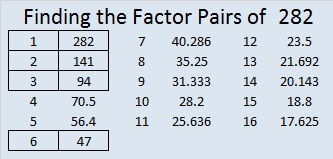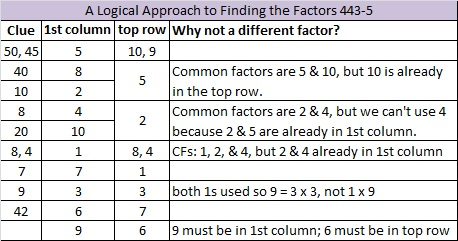282 and Level 5

• 282 is a composite number.
• Prime factorization: 282 = 2 x 3 x 47
• The exponents in the prime factorization are 1, 1, and 1. Adding one to each and multiplying we get (1 + 1)(1 + 1)(1 + 1) = 2 x 2 x 2 = 8. Therefore 282 has 8 factors.
• Factors of 282: 1, 2, 3, 6, 47, 94, 141, 282
• Factor pairs: 282 = 1 x 282, 2 x 141, 3 x 94, or 6 x 47
• 282 has no square factors that allow its square root to be simplified. √282 ≈ 16.793Today is Halloween. Give this Level 5 puzzle a try. . . Trick or Treat?Print the puzzles or type the factors on this excel file: 10 Factors 2014-10-27